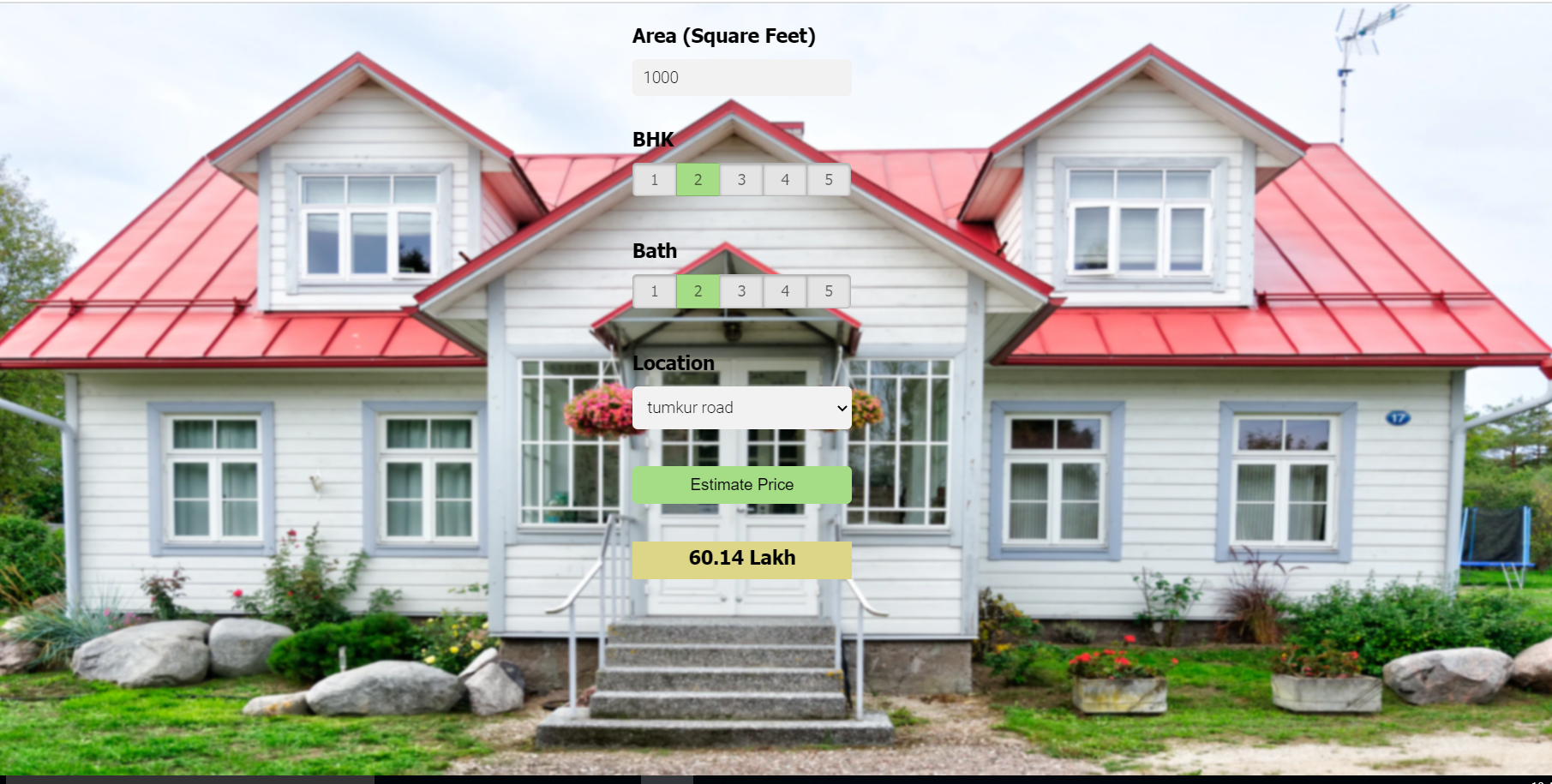# How ML work for house price prediction part-2

Original article was published by Jigar prajapati on Artificial Intelligence on Medium# How ML work for house price prediction part-2

This Data science use case walks through step by step process of how build a house price prediction.

Some technology used for this model is 1) python 2) Numpy and pandas 3) Matplotlib 4) Sklearn

For This model Bengaluru House price datadataset is used from kaggle

Lets start with data, this dataset contain some basic information of houses like area type, location, size, price, etc…

First step is we remove unnecessary data from this data set which no affect on price estimate like area_type, society, balcony and availability. so after removing that data our dataset is

Now we start with Data cleaning process, we check is any null values in dataset or not? So, there is no null values in dataset. that make work so easy.

check the float values in total_sqft like 2100–2850, we have to convert this float datatype into integer datatype

now split the size features data with space and make new feature called BHK which contain number of room in house.

This is how we convert float into integer number.

now let’s do some EDA and make new feature using price and total_sqft .

“dimensionality reduction” having to many values in location might affect the model, so we reduce it.

make a function which contain all the location having less than 10 state and replace with “other” .

“Remove outliers” Here we find that min price per sqft is 267 rs/sqft whereas max is 176470, this shows a wide variation in property prices. We should remove outliers per location using mean and one standard deviation

Let’s check if for a given location how does the 2 BHK and 3 BHK property prices look like

We should also remove properties where for same location, the price of (for example) 3 bedroom apartment is less than 2 bedroom apartment (with same square ft area). What we will do is for a given location, we will build a dictionary of stats per bhk, i.e.

{ ‘1’ : { ‘mean’: 4000, ‘std: 2000, ‘count’: 34 }, ‘2’ : { ‘mean’: 4300, ‘std: 2300, ‘count’: 22 },
}

create a model using linear regression.

Use K Fold cross validation to measure accuracy of our Linear Regression model

Find best model using GridSearchCV

Make function to use model and Test the model for few properties.

# This is how price estimate model work in real estate business.

Motivation:- codebasics